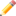This article is a stub. You can help Programmer's Wiki by expanding it.Remember to remove this when the stub is fixed.

## Terminology

• vertex - points on a graph. aka node
• edge - connection between two vertices.

## Data Structures

Graphs can be represented in several ways. An adjacency list is a list of the graph's vertices. each vertex then has a list of the vertices it is connected to. An adjacency matrix stores edges in a matrix. The rows and columns represent the vertices in the graph. If there is an edge between two vertices, a one is placed at their intersection. the absence of an edge is shown with a zero at that position.

## Operations

There are many algorithms that have been created for handling graphs. For searching graphs depth-first search or breadth-first search can be used. Dijkstra's algorithm to find the shortest path from one node to another.